Informacje o czasopiśmieFormat
Czasopismo
eISSN
2300-0929
Pierwsze wydanie
19 Oct 2012
Częstotliwość wydawania
4 razy w roku
Języki
AngielskiOtwarty dostęp

# Sensitivity of Aerodynamic Characteristics of Paraglider Wing to Properties of Covering Material

###### Zakres stron:64 - 72
Informacje o czasopiśmieFormat
Czasopismo
eISSN
2300-0929
Pierwsze wydanie
19 Oct 2012
Częstotliwość wydawania
4 razy w roku
Języki
Angielski

The paper is theoretically oriented. The main goal is to analyze the sensitivity of aerodynamic characteristics to the properties of the material used for paraglider wing. The paraglider of considerable dimensions is designed without stiffening elements. Thus, the covering material yields adequate pressure distribution between the external and internal parts of the wing. The problem is solved using a geometrical model approximated by the dimensionless coordinates of crucial points and smoothed by spline curves. The finite volume mesh is defined using the Ansys Meshing program. Numerical analysis uses five different covering materials, ranging from the air-impermeable covering to the covering subjected to hydrolytic—mechanical degradation. Optimization of properties of the covering material improves the lift force and the aerodynamic characteristics of the wing. Moreover, numerical modeling is more beneficial and efficient than prototype tests. The obtained pressure distributions and other parameters explain the aerodynamic safety of the paraglider during dynamic conditions of flight.

#### Keywords

Introduction

The paraglider wing is a flexible structure without stiffening elements of the elliptical contour in the top view and a cross-sectional airfoil profile during the flight. It acquires the aerodynamic shape due to the air pressure entering the vents on the leading edge of the wing. The covering is made of impregnated synthetic textiles, which (i) reduces the material’s permeability coefficient; (ii) influences the cl/cd ratio; (iii) creates the lift force by the advantageous pressure difference under dynamic conditions of the flight, i.e., the negative pressure on the upper plane and the overpressure on the lower surface of the wing.

The textile covering should be lightweight, shock- and ultraviolet (UV)-resistant, and waterproof. The impregnated synthetic textiles of reduced permeability coefficient are made of nylon PA 6.6 [1, 2], which results in an increased cl/cd ratio, i.e., better aerodynamic performance. The uniform impregnated layer of dimensional tolerance of 10−3 m improves the air permeability. Although its composition is not announced by manufacturers, the impregnant is usually based on polyurethane (PU). Fabrics are manufactured using the Ripstop technology, i.e., the reinforced threads are located at regular distances in the square form .

The problem of wing dynamics during the flight is defined by a state variable, which is the pressure. The fundamentals concerning paragliders and the mechanics of flight can be found elsewhere [4, 5]. The problem is solved using a geometrical model of the structure approximated by dimensionless coordinates of crucial points and smoothed by spline curves. The physical model is defined using the geometrical model, finite volume method, and accompanying phenomena. It is determined by the flight parameters (the lift force, the drag force, and the cl/cd ratio), the surrounding conditions, and so on. The mathematical model introduces a set of differential equations accompanied by the boundary conditions [6, 7, 8]. The problem is solved numerically by applying the wing model in Ansys program. The distribution of state variables can be visualized using any graphical program . This paper is a continuation of previous work .

The main goal of the article is to determine the sensitivity of aerodynamic characteristics to the properties of the covering material of a paraglider wing. A set of different materials is introduced, ranging from the air-impermeable one to that subjected to hydrolytic–mechanical degradation. Optimization of the material properties improves the lift force and the aerodynamic characteristics of the paraglider.

The novel elements are the following. (i) The available literature does not introduce the effect of material permeability on the aerodynamic characteristics of the wing. (ii) A set of parameters determining the aerodynamic characteristics of the paraglider are introduced. (iii) The obtained pressure distributions explain the aerodynamic safety of the paraglider in dynamic conditions.

Geometrical model of the Paraglider wing

Let us first assume a universal paraglider wing of improved sliding capacity, and its total weight reduced to 5 kg. To determine the geometrical model, the wing contour and airfoil cross section of the real structure were measured. The initial step is to determine the 23 dimensionless coordinates creating the airfoil profile within the XZ plane (Figure 1). Next, the geometry of the wing contour is defined in the XY plane (Figure 2). The coordinates of the particular point in the 3D Cartesian coordinate system are calculated using the elliptic equation in the following form: $x=xiCj+12(Co−cj);y=(R+zjCj)sin(yjR);z=[(R+zjCj)cos(yjR)]−R$ x = {x_i}{C_j} + {1 \over 2}\left( {{C_o} - {c_j}} \right);y = \left( {R + {z_j}{C_j}} \right)\sin \left( {{{{y_j}} \over R}} \right);z = \left[ {\left( {R + {z_j}{C_j}} \right)\cos \left( {{{{y_j}} \over R}} \right)} \right] - R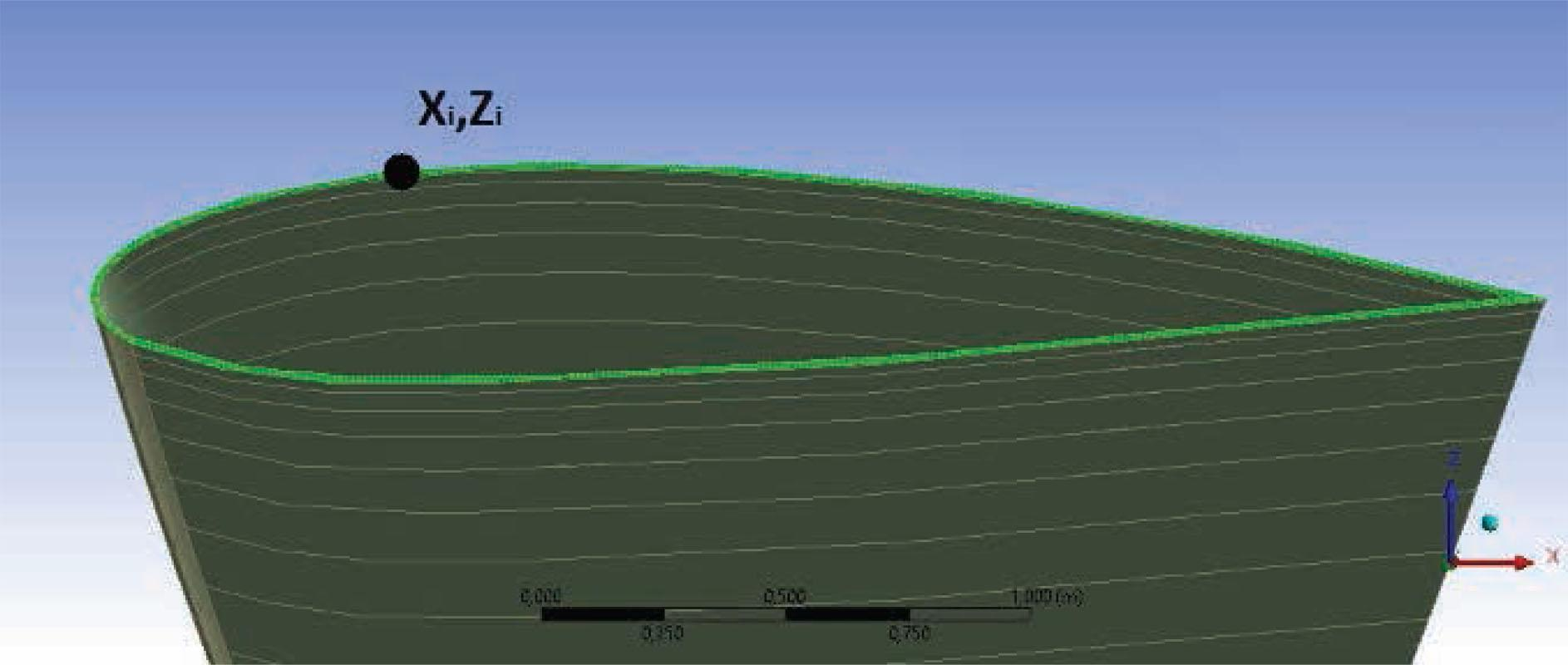Parameters characterizing the dimensionless coordinates of the wing profile. Xi; Zi dimensionless coordinates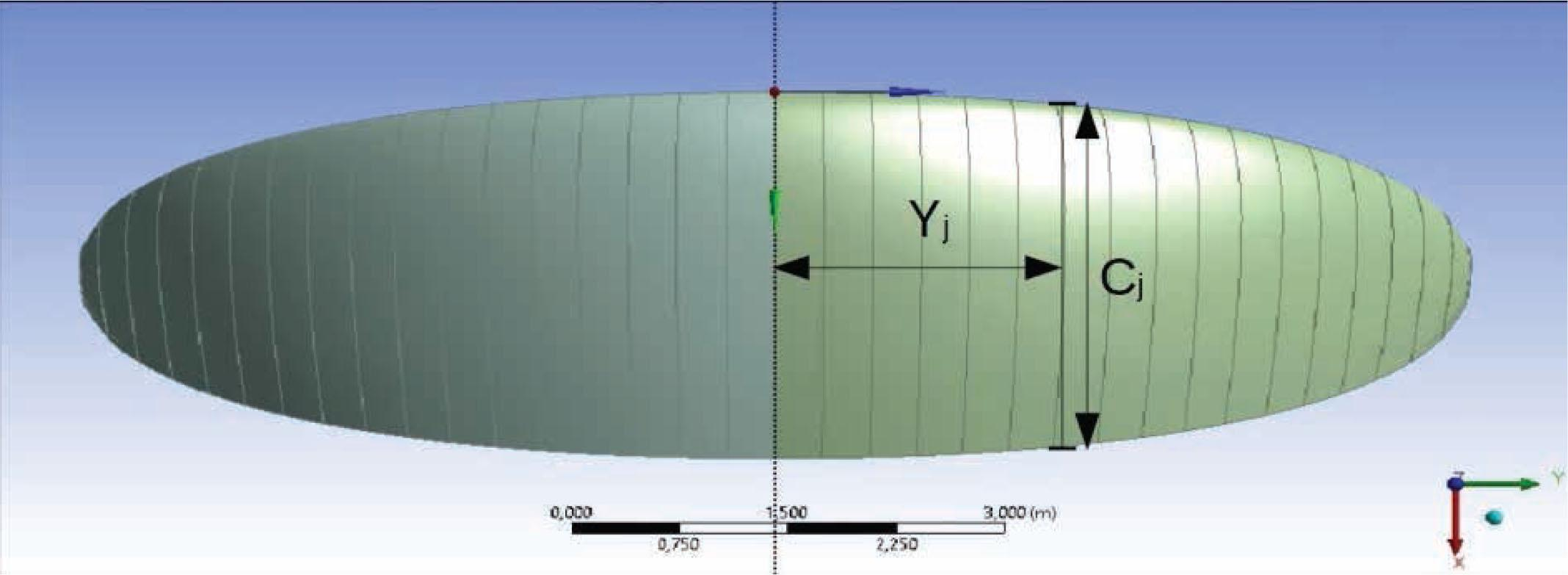Parameters characterizing the elliptical contour of the wing profile Yi – distance between the j-th rib and the symmetry plane, Cj – length of the j-th rib

The coordinates can be introduced into the simple numerical program in MatLab environment to create the coordinates of an optional point on the wing contour. These points are connected by the smoothing curves of the spline type to create the ribs’ profile. The final contour is obtained by incorporating the material surface for these points of assumed thickness of 2 × 10−3 m, which is the typical value for the paraglider (Figure 3).

Let us also assume a set of covering materials (Table 1). The basic material is always made of the polyamide PA 6.6 of plain weave using the Ripstop reinforcement. The air-impermeable material is a theoretical one, which limits the flight characteristics.

Characteristics of materials selected to numerical modeling.

Parameter tested SKYTEX 27 CLASSIC 2 PORCHER MARINE 9017 PU E38A SKYTEX 27 CLASSIC after 7 washings, each (1h 30°C) SKYTEX 36 hydrolytic-mechanically degradated Air-impermeable material (theoretical)
Surface mass g/m2 29 40 24 34 -
Air-permeability under pressure 2000 Pa l/m2/min 3 40 120 200 0
Coverage one-sided, double, PU one-sided, single, PU one-sided, single, PU one-sided, single, PU
Number of threads 1/cm warp: 70 warp: 60 warp: 70 warp: 65 -
weft: 70 weft: 60 weft:70 weft: 65 -
Creation of finite volume mesh in Ansys Meshing program

The geometrical model of a paraglider is introduced into the finite volume mesh of cuboid elements. Due to its symmetry, only a half of the structure is considered using the condition Symmetry. The dimensions of the control volume are 20 m in front of the wing and 40 m in other directions. The external surfaces of the cuboid control volume are defined by the boundary condition Pressure Far-Field, which is the undisturbed flow of the prescribed values of velocity, pressure, and temperature of the surrounding air. The finite volume mesh is created by standard procedures.

Sweep method

First, the selected points around the wing surface are created. Next, the mesh topology is extended in the source plane (wing surface) along the object to create the surface mesh of prescribed density. The last step is the segmentation along the planes perpendicular to the source plane, which is divided into 80 elements.

Tetrahedron patch conforming method

The procedure creates a finite volume mesh for the medium (the air) in the surrounding space.

Edge sizing

This method of mesh dimensioning introduces the prescribed number of nodes on the selected edges/segments. Each part of the upper and lower profiles is divided into 30 parts, whereas the short profile of air inlet is divided into three parts.

Face sizing

This method of mesh dimensioning incorporates the prescribed dimensions of each element on the selected surfaces. The procedure is applied to model the air inside the paraglider in the plane of symmetry.

The finite volume mesh of 474264 elements is generated using the Ansys Meshing program (Figure 4). The mesh quality is relatively good because the mean value of the parameter Orthogonal Quality is equal to 0.7629 (the perfect mesh has Orthogonal Quality = 1) and Skewness is 0.2463 (the perfect mesh has Skewness = 0). The deviations are absolutely acceptable for the considered number of the finite volume mesh.

Numerical solutions

The problem was solved in the Ansys Fluent environment applying the Spalart–Allmaras turbulence model. The fluid filling the computational volume is air, which is determined by the procedure Materials; air → ideal gas. Thus, the boundary condition Pressure Far-Field can be applied on each surface of the cuboid (except the plane of symmetry). The outer surfaces are at sufficient distance from the examined object; therefore, they might present a state of undisturbed flow. Thus, the parameters of the surroundings are the following: temperature T = 300 K; pressure p = 101325 Pa; velocity v = 0.037 Ma; and angle of attack a = 6°. The plane of symmetry is described by the condition Symmetry. The boundary condition given to the walls creating the wing surface is Interior; this condition enabled the use of the model Porous Media (Cell Zone Conditions → Porous Media) for the cells creating the paraglider wing geometry. Therefore, the materials are introduced into the numerical model using the effective air permeability. The corresponding condition Porous Resistance has the following form: $1α=−Δptμvm$ {1 \over \alpha } = - {{\Delta p} \over {t\mu {v_m}}}

The parameter Porous Resistance is determined in Table 2, for porous materials transmitting air according to Table 1, except for the air-impermeable covering.

Parameter Porous Resistance for different coating materials.

Parameter tested SKYTEX 27 CLASSIC 2 PORCHER MARINE 9017 PU E38A SKYTEX 27 CLASSIC after 7 washings, each (1h 30°C) SKYTEX 36 hydrolytic-mechanically degradated
Air-permeability under pressure 2000 N l/m2/min 3 40 120 200
Velocity ·10−4 m/s 50 67 200 333
1/α 1/m2 1.118 ▪ 1015 8.385 ▪ 1013 2.795 ▪ 1013 1.677 ▪ 1013

The dynamics of air motion is defined by the conservation equations of mass, momentum, and energy in vector form, which can be simplified to the set of equivalent scalar equations. The corresponding differential equations are accompanied by the boundary conditions, i.e., the environmental conditions. $∭V∂ρ∂tdV+∬AρvndA=0ddt∭Vρv→dV=∬Ap→ndA+∭VF→mρdVddt∭Vρ(cwT+v22)dV=∬Ap→n⋅v→dA+∭VρF→m⋅v→dV+∬Aq˙nρA+∭Vq˙mρdV$ \matrix{ {\mathop{\int\!\!\!\int\!\!\!\int}\limits_{\kern-5.5pt V} {{{{\partial _\rho }} \over {{\partial _t}}}dV + \int\!\!\!\int\limits_A {\rho {v_n}dA = 0} } } \hfill \cr {{d \over {dt}}\mathop{\int\!\!\!\int\!\!\!\int}\limits_{\kern-5.5pt V} {\rho \vec vdV} = \int\!\!\!\int\limits_A {{{\vec p}_n}dA} + \mathop{\int\!\!\!\int\!\!\!\int}\limits_{\kern-5.5pt V} {{{\vec F}_m}\rho dV} } \hfill \cr {{d \over {dt}}\mathop{\int\!\!\!\int\!\!\!\int}\limits_{\kern-5.5pt V} {\rho \left( {{c_w}T + {{{v^2}} \over 2}} \right)dV = \int\!\!\!\int\limits_A {{{\vec p}_n} \cdot \vec vdA} + \mathop{\int\!\!\!\int\!\!\!\int}\limits_{\kern-5.5pt V} {\rho {{\vec F}_m} \cdot \vec vdV} + \int\!\!\!\int\limits_A {{{\dot q}_n}dA} + } \int\!\!\!\int\!\!\!\int_V {{{\dot q}_m}\rho dV} } \hfill }

The aerodynamic force is generated by an advantageous pressure distribution, i.e., negative pressure on the upper plane and overpressure on the lower surface, and it has the following form: $F=clSρv22$ F = {c_l}S\rho {{{v^2}} \over 2}

The resultant aerodynamic force during the flight can be decomposed into the advantageous lift force L and the drag force D. The greater the lift force compared to the drag force, the greater is the cl/cd ratio, according to the following formula : $cl/cd=LD$ {c_l}/{c_d} = {L \over D}

Results and discussion
Air-impermeable material – the comparative material

First, a low Courant number (C = 2) is introduced; this is the main control parameter for the stability of the calculation procedure. It defines the time step size (for the given cell geometry and velocity through the cell). The exact expression binding the time step size and Courant number is very complex. However, the unidirectional flow along the X-axis is determined by the simplified equation in the following form: $Δt=CΔxU$ \Delta t = {{C\Delta x} \over U}

Because the calculations are stable, the Courant number is increased to the value 15, and the time step is considerably increased. The assumed number of iterations is 900.

The obtained mean pressure inside the wing contour is equal to 101415.83 Pa; the mass flow rate is 0 kg/s; the lift force is 1101.96 N; the drag force is 83.6805 N; and the cl/cd ratio is 13.1687.

The pressure distribution in the plane of symmetry is uniform, and the mean value is considerably greater than the surrounding pressure, which yields good aerodynamic properties during the flight (pressure distribution comparable to the view presented in Figure 5). The pressure on the upper surface of the paraglider wing is maximal on the leading edge and decreases by 150 Pa to the value of 101230 Pa at a distance of 0.5 m from the leading edge. On the lower surface, the pressure is more stable and decreases by 15 Pa to the value of 101315 Pa at a distance of 0–0.5 m from the leading edge. Both distributions yield the favorable configuration of overpressure and negative pressure on both respective wing surfaces.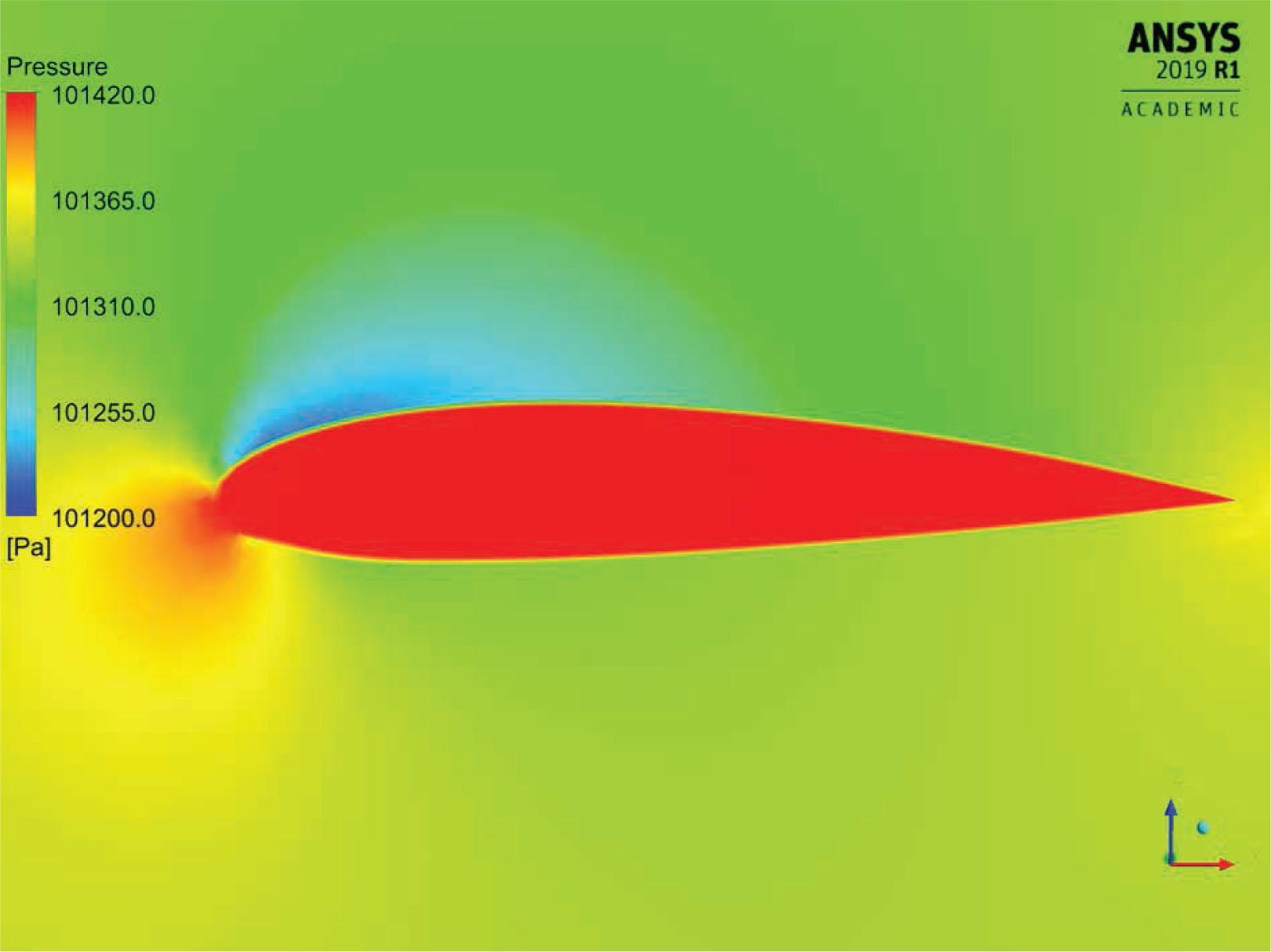Pressure distribution in symmetry plane, representative for cases of good aerodynamic characteristics (wing made of SKYTEX 27 CLASSIC 2 material)
Material Skytex 27 Classic 2 (air permeability 3 L/m2/min)

The calculations are relatively unstable, and the assumed number of iterations increases to 2000. The obtained mean pressure inside the wing is 101415.80 Pa; the mass flow rate is 0.1280 kg/s; the lift force is 1093.99 N; the drag force is 85.6634 N; and the cl/cd ratio is 12.7708.

The aerodynamic characteristics and the pressure distributions are comparable with the values obtained for the impermeable material (Figures 5 and 7). The air permeability loss is negligible and does not influence the pressure distribution on both wing surfaces.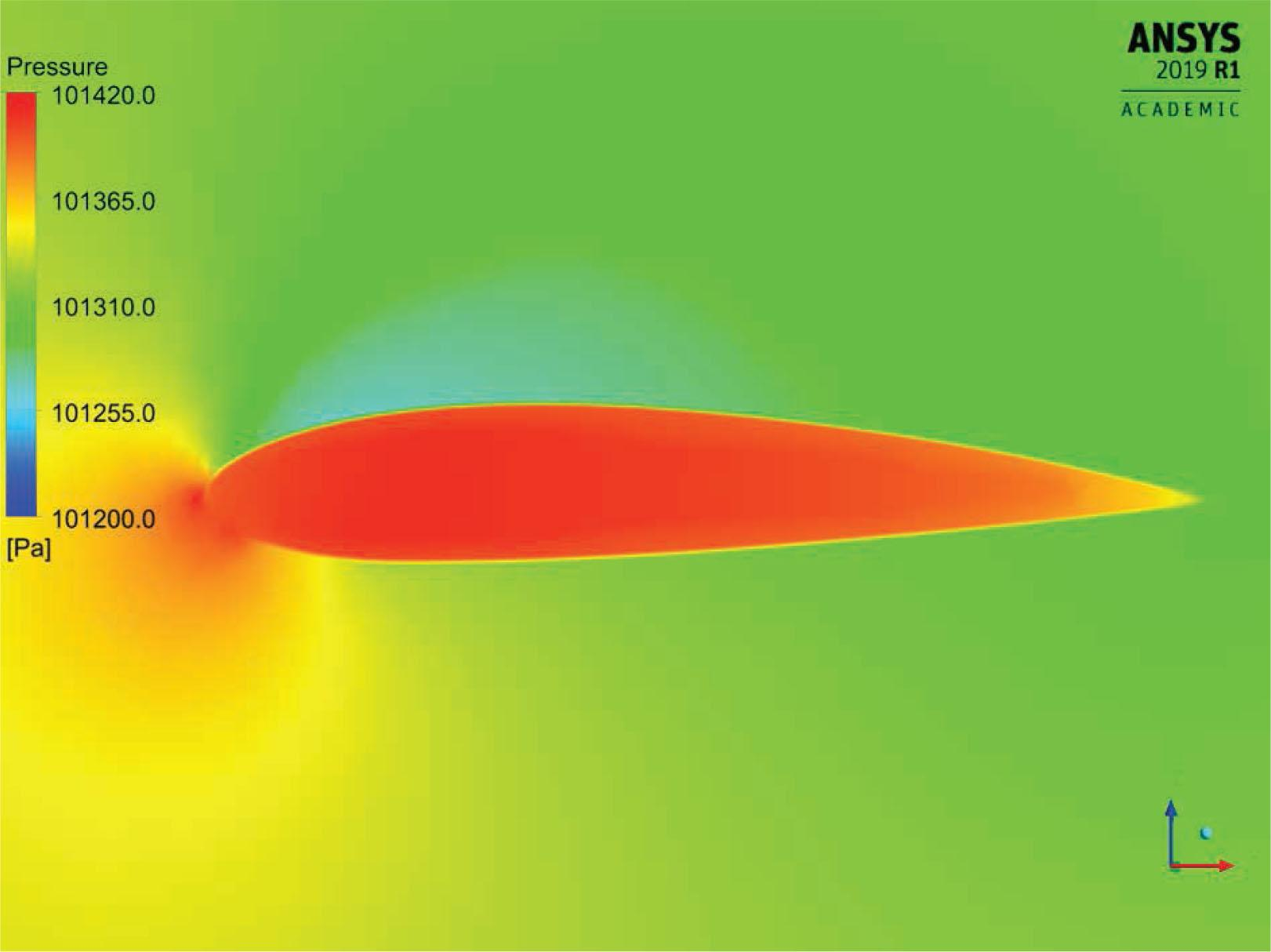Pressure distribution in symmetry plane, representative for cases of unfavorable pressure distribution (wing made of SKYTEX 36 hydrolytic-mechanically degradated material)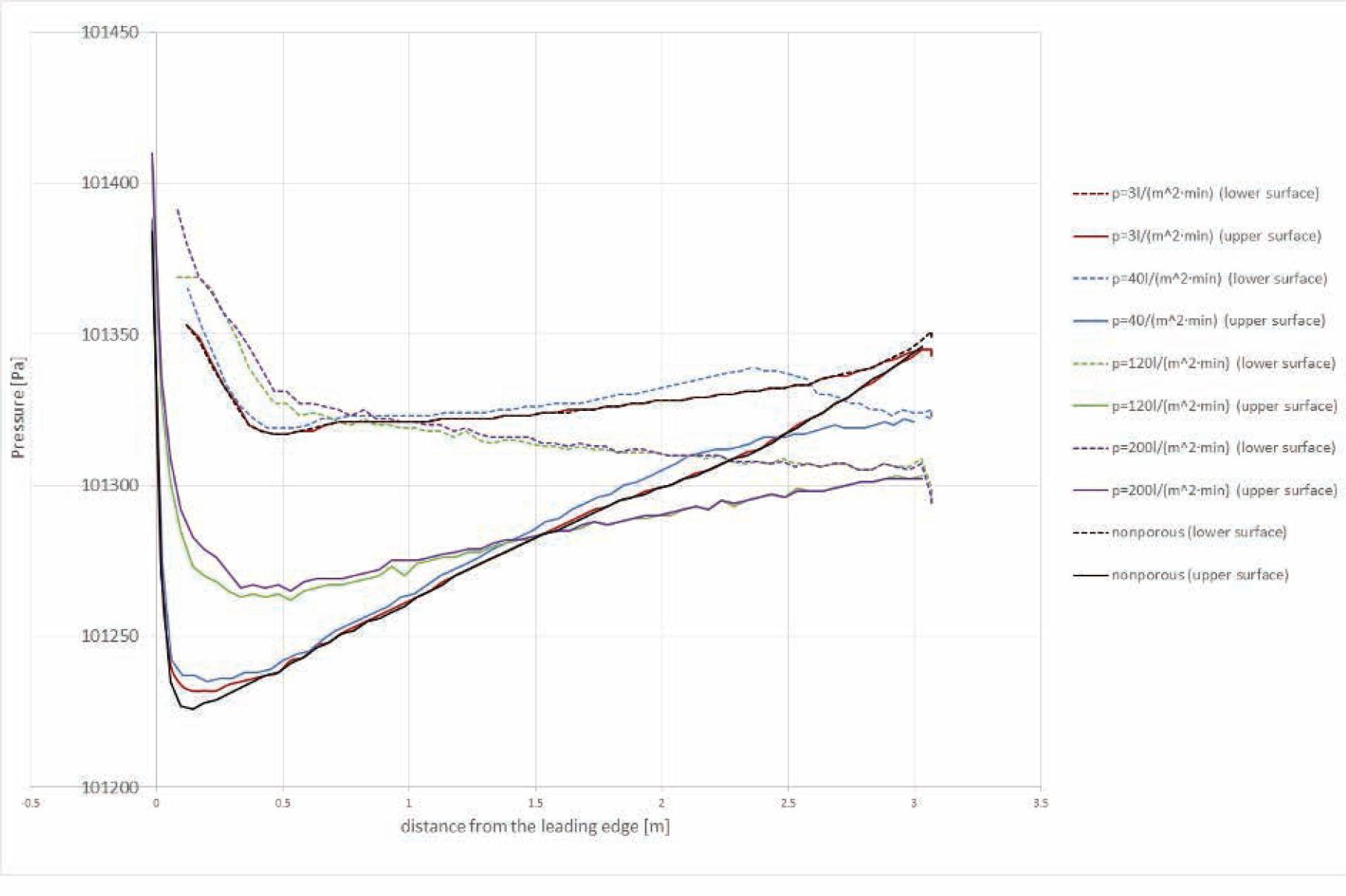Pressure on upper surfaces (solid lines) and lower surfaces (dotted line) of wing surfaces considering each case according to the legend
Material Porcher Marine 9017 PU E38A (air permeability 40 L/m2/min)

The calculations are slightly unstable, and the assumed number of iterations is 2000. The obtained mean pressure inside the paraglider is 101412.60 Pa; the mass flow rate is 1.435 kg/s; the lift force is 1085.883 N; the drag force is 93.4068 N; and the cl/cd ratio is 11.6253.

Although the wing has satisfactory aerodynamic characteristics, some values change for the worse. The cl/cd ratio, which is equal to 11.6253, decreases by 1.5434 compared to the impermeable material. The mean pressure inside the paraglider is also decreased. The wing is still safe and stable during the flight and has acceptable pressure distribution inside the airfoil profile.

The pressure drop above the wing is less significant than before, but it is sufficient to create the aerodynamic force. The overpressure inside the wing significantly exceeds the pressure in the entire calculation area and is evenly distributed. The pressure distribution can be compared to the view presented in Figure 5. It follows that the wing will be characterized by stable flight, even with light blasts of wind.

According to Figure 7, the pressure on the upper surface increases rapidly to 101400 Pa at the leading edge, then rapidly decreases to 101235 Pa at a distance of 0–0.5 m from the leading edge. The pressure drop on the bottom surface is much smaller and equal to 10 Pa compared to the surrounding pressure. There are some cases of instability at the distances of 2.5 m from the leading edge on the upper surface and 0.5 m on the bottom surface because the pressure curves are almost horizontal. Thus, the courses stabilize rapidly and achieve atmospheric pressure at the trailing edge.

Material Skytex 27 Classic after seven washings (air permeability 120 L/m2/min)

The calculations are relatively unstable, and the number of iterations is 2000. The obtained mean pressure inside the paraglider is 101409.11 Pa; the mass flow rate is 3.5574 kg/s; the lift force is 812.5661 N; the drag force is 187.2534 N; and the cl/cd ratio is 4.3394.

The aerodynamic force reaches much lower values: the area of negative pressure above the wing is considerably smaller than in the previous cases. The high value of pressure outside the wing is dangerous with respect to the mean pressure inside the structure, particularly close to the leading edge. The pressure distribution can be compared to the view presented in Figure 6. The dynamic conditions during the flight could generate flight instability as follows: (i) the disturbance of the wing shape because of low intrinsic stiffness; (ii) the abrupt disturbance of aerodynamic characteristics, as even a slightly stronger blast of wind could result in a collapse. The pressure inside the wing close to the leading edge is much lower than the mean pressure inside the paraglider (around 101385 Pa). Thus, it is difficult to control and operate this paraglider; it may endanger the user’s health or life.

The negative pressure above the wing reaches the extreme value at a distance of 0.25–0.5 m from the leading edge (Figure 7). The pressure drop is insignificant, and the pressure difference on the upper and lower surfaces at a distance of 0.25–0.5 m from the leading edge is about 65 Pa. Compared to the wing made of impermeable material, the difference is about 90 Pa. Starting from a distance of 0.5 m from the leading edge, the pressure at the lower and upper surfaces begins to stabilize; at the trailing edge, the pressure reaches 101295 Pa and is about 30 Pa lower than atmospheric air pressure. For a paraglider made of air-impermeable material, the pressure at the trailing edge is higher than the air pressure at a large distance from the object. The pressure distribution on the wing surfaces additionally confirms the flight instability.

Material Skytex 36 subjected to hydrolytic–mechanical degradation (air permeability 200 L/m2/min)

The calculations are relatively unstable, and the number of iterations is 2000. The obtained mean pressure inside the paraglider is 101398.35 Pa; the mass flow rate is 3.8542 kg/s; the lift force is 812.2437 N; the drag force is 199.7884 N; and the cl/cd ratio is 4.0655.

The mean pressure inside the paraglider is much lower than in the previous cases, which generates an insignificant lift force (Figure 6). The internal pressure at the leading edge is higher than the mean pressure inside the wing. Therefore, it would be probably impossible to fly, because the paraglider is made of material with a slight intrinsic stiffness subjected to very high pressure at the leading edge. The overpressure inside the wing is insufficient to create the stable aerodynamic shape, and hence, the paraglider analyzed is extremely dangerous.

As before, a rapid increase in pressure on the leading edge is observed; it reaches about 101405 Pa, while the average pressure inside the paraglider is 101398.35 Pa (Figure 7). The pressure difference on the upper and lower surfaces at a distance of about 0.5 m from the leading edge is about 60 Pa. The pressure at the trailing edge reaches lower values than the atmospheric pressure, and the paraglider is extremely unstable and hazardous.

Question of verification

The paper is theoretically oriented. Modeling and optimization of aerodynamic characteristics and properties of the covering material are significant for a paraglider structure, being more beneficial and efficient than prototype tests. The wing of considerable dimensions and complicated structure is designed without stiffening and is manufactured in specialized factories. The costs of the materials, finishing procedures, production, and amount of work are significant.

The validation of results for the complete paraglider is impossible because of the expensive manufacturing process of the prototype. The tests are implementable inside a large-size wind tunnel, which is inaccessible in Poland. The dynamic conditions of flight will be now extremely dangerous for the pilot.

Modeling on a reduced scale is unrealizable because of the nonexistence of manufacturing technology for reduced dimensions and due to the high manufacturing costs. The price of the scaled-down prototype is much higher than the paraglider on a 1:1 scale. The structure of reduced dimensions has stiffness during the flight uncompared to the real object and is hard to operate (the pilot has standard dimensions).

Thus, only a theoretical model enables the analysis of the global sensitivity of the paraglider characteristics to different materials, optimization of the pressure distribution inside and outside the contour, and optimization of the structural shape. Some parameters can be partially verified, e.g., the air permeability and creation of the single airfoil profile. However, the problem is complicated and beyond the scope of the paper presented and will be discussed in a consecutive article.

Conclusions

Numerical analysis considers five different covering materials and a few parameters determining the aerodynamic characteristics, viz., the mean pressure inside the wing profile, the mass flow rate, the aerodynamic force, the lift force, the drag force, the cl/cd ratio, the pressure distributions in the plane of symmetry, and the pressure distribution on the upper and lower wing surfaces.

The best aerodynamic characteristics are presented by the paraglider made of air-impermeable covering (the comparative material). The pressure inside the wing contour is 101415.83 Pa; and aerodynamic excellence is 13.1687. However, the paraglider made of material of higher air permeability (3 L/m2/min) presents comparable properties. Its aerodynamic excellence decreased by only 0.3979. That is, assuming a starting height of 1 km, the wing of better characteristics reaches a distance longer by 388 m during the gliding flight. The analysis does not include the additional resistance of the rigging and the pilot attached in the harness.

The paraglider made of Porcher Marine 9017 PU E38A material (used approximately 20 years ago) has much worse parameters than the previous two structures. Its cl/cd ratio reaches 11.6253, although the wing still generates sufficient aerodynamic force. Based on the pressure distribution, the wing covered by this material is safe and stable during the flight.

The last two materials are degraded and show the worst characteristics. The paraglider made of Skytex 27 Classic material after seven washes is usable but dangerous. The wing covered by Skytex 36 fabric subjected to hydrolytic–mechanical degradation is probably unfit for flight. A very high pressure at the leading edge disturbs and creates overpressure inside the wing and forms an aerodynamic shape. The flight is extremely dangerous.

Summarizing, the aerodynamic characteristics of paragliders covered by the currently used fabrics are comparable with those of paragliders covered by air-impermeable material. The technological progress yields adequate wing covering; the materials have improved air permeability and lower surface masses.

The textile covering can be also optimized with respect to the reduced surface mass of acceptable strength. The air permeability, material strength, and susceptibility to permanent deformation should be improved. The other question is the resistance to damage caused by fabric aging and finishing procedures. These characteristics can be next optimized in terms of the coupled heat and moisture transport within the covering material [7, 10, 11].

#### Parameter Porous Resistance for different coating materials.

Parameter tested SKYTEX 27 CLASSIC 2 PORCHER MARINE 9017 PU E38A SKYTEX 27 CLASSIC after 7 washings, each (1h 30°C) SKYTEX 36 hydrolytic-mechanically degradated
Air-permeability under pressure 2000 N l/m2/min 3 40 120 200
Velocity ·10−4 m/s 50 67 200 333
1/α 1/m2 1.118 ▪ 1015 8.385 ▪ 1013 2.795 ▪ 1013 1.677 ▪ 1013

#### Characteristics of materials selected to numerical modeling.

Parameter tested SKYTEX 27 CLASSIC 2 PORCHER MARINE 9017 PU E38A SKYTEX 27 CLASSIC after 7 washings, each (1h 30°C) SKYTEX 36 hydrolytic-mechanically degradated Air-impermeable material (theoretical)
Surface mass g/m2 29 40 24 34 -
Air-permeability under pressure 2000 Pa l/m2/min 3 40 120 200 0
Coverage one-sided, double, PU one-sided, single, PU one-sided, single, PU one-sided, single, PU
Number of threads 1/cm warp: 70 warp: 60 warp: 70 warp: 65 -
weft: 70 weft: 60 weft:70 weft: 65 -

Anonymous, http://www.porcher-sport.com/fr/. Anonymous http://www.porcher-sport.com/fr/ Search in Google Scholar

Anonymous, https://www.porcher-ind.com/en. Anonymous https://www.porcher-ind.com/en Search in Google Scholar

Maślanka, P., Korycki, R. (2019). Textile cover effect on aerodynamic characteristics of a paraglider wing. Fibres Textiles in Eastern Europe, 27,133(1), 78–83. MaślankaP. KoryckiR. 2019 Textile cover effect on aerodynamic characteristics of a paraglider wing Fibres Textiles in Eastern Europe 27, 133 1 78 83 10.5604/01.3001.0012.7511 Search in Google Scholar

Dudek, P., Włodarczak, Z. (2013). Paragliding (in Polish). Arete. DudekP. WłodarczakZ. 2013 Paragliding (in Polish) Arete. Search in Google Scholar

Krzyżanowski, A. (2009). Mechanics of flight (in Polish). WAT, Warsaw. KrzyżanowskiA. 2009 Mechanics of flight (in Polish) WAT Warsaw Search in Google Scholar

Korycki, R. (2001). Two-dimensional shape identification for the unsteady conduction problem. Structural and Multidisciplinary Optimization, 21(4), 229–238. KoryckiR. 2001 Two-dimensional shape identification for the unsteady conduction problem Structural and Multidisciplinary Optimization 21 4 229 238 10.1007/s001580050187 Search in Google Scholar

Korycki, R. (2009). Method of thickness optimization of textile structures during coupled heat and mass transport. Fibres and Textiles in Eastern Europe, 17,1(72), 33–38. KoryckiR. 2009 Method of thickness optimization of textile structures during coupled heat and mass transport Fibres and Textiles in Eastern Europe 17, 1 72 33 38 Search in Google Scholar

Korycki, R., Szafrańska, H. (2013). Modelling of temperature field within textile inlayers of clothing laminates. Fibres Textiles in Eastern Europe, 214(100), 118–122. KoryckiR. SzafrańskaH. 2013 Modelling of temperature field within textile inlayers of clothing laminates Fibres Textiles in Eastern Europe 214 100 118 122 Search in Google Scholar

Puszkarz, A. K., Korycki, R., Krucinska, I. (2016). Simulations of heat transport phenomena in a three-dimensional model of knitted fabric. Autex Research Journal, 16(3), 128–137. PuszkarzA. K. KoryckiR. KrucinskaI. 2016 Simulations of heat transport phenomena in a three-dimensional model of knitted fabric Autex Research Journal 16 3 128 137 10.1515/aut-2015-0042 Search in Google Scholar

Korycki, R., Szafrańska, H. (2016). Optimisation of pad thicknesses in ironing machines during coupled heat and mass transport. Fibres and Textiles in Eastern Europe, 24, 1(115), 128–135. KoryckiR. SzafrańskaH. 2016 Optimisation of pad thicknesses in ironing machines during coupled heat and mass transport Fibres and Textiles in Eastern Europe 24, 1 115 128 135 10.5604/12303666.1172095 Search in Google Scholar

Korycki, R., Szafranska, H. (2016). Thickness optimisation of textiles subjected to heat and mass transport during ironing. Autex Research Journal, 16(3), 165–174. KoryckiR. SzafranskaH. 2016 Thickness optimisation of textiles subjected to heat and mass transport during ironing Autex Research Journal 16 3 165 174 10.1515/aut-2015-0035 Search in Google Scholar

• #### Modeling of Material Characteristics of Conventional Synthetic Fabrics

Polecane artykuły z Trend MD